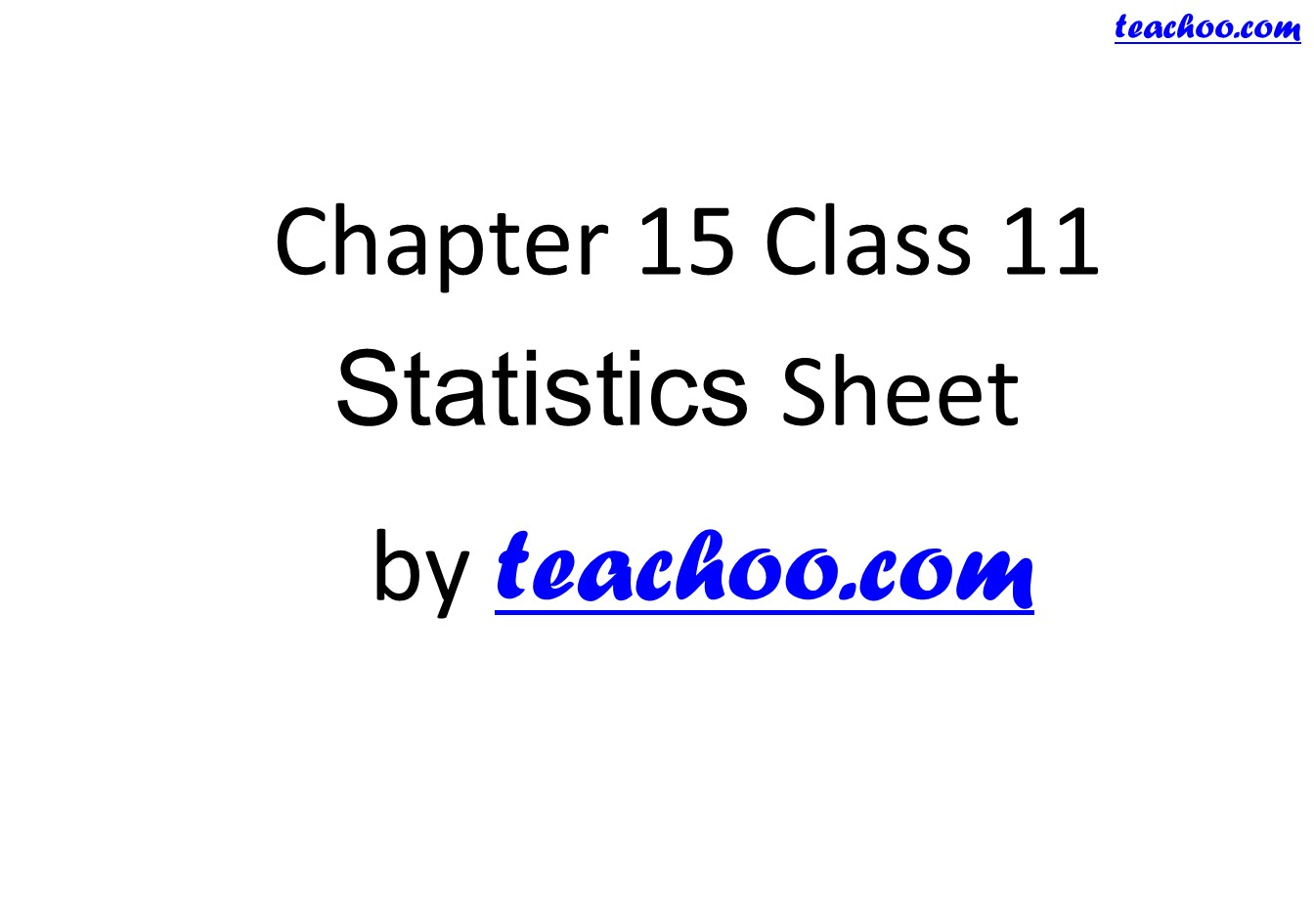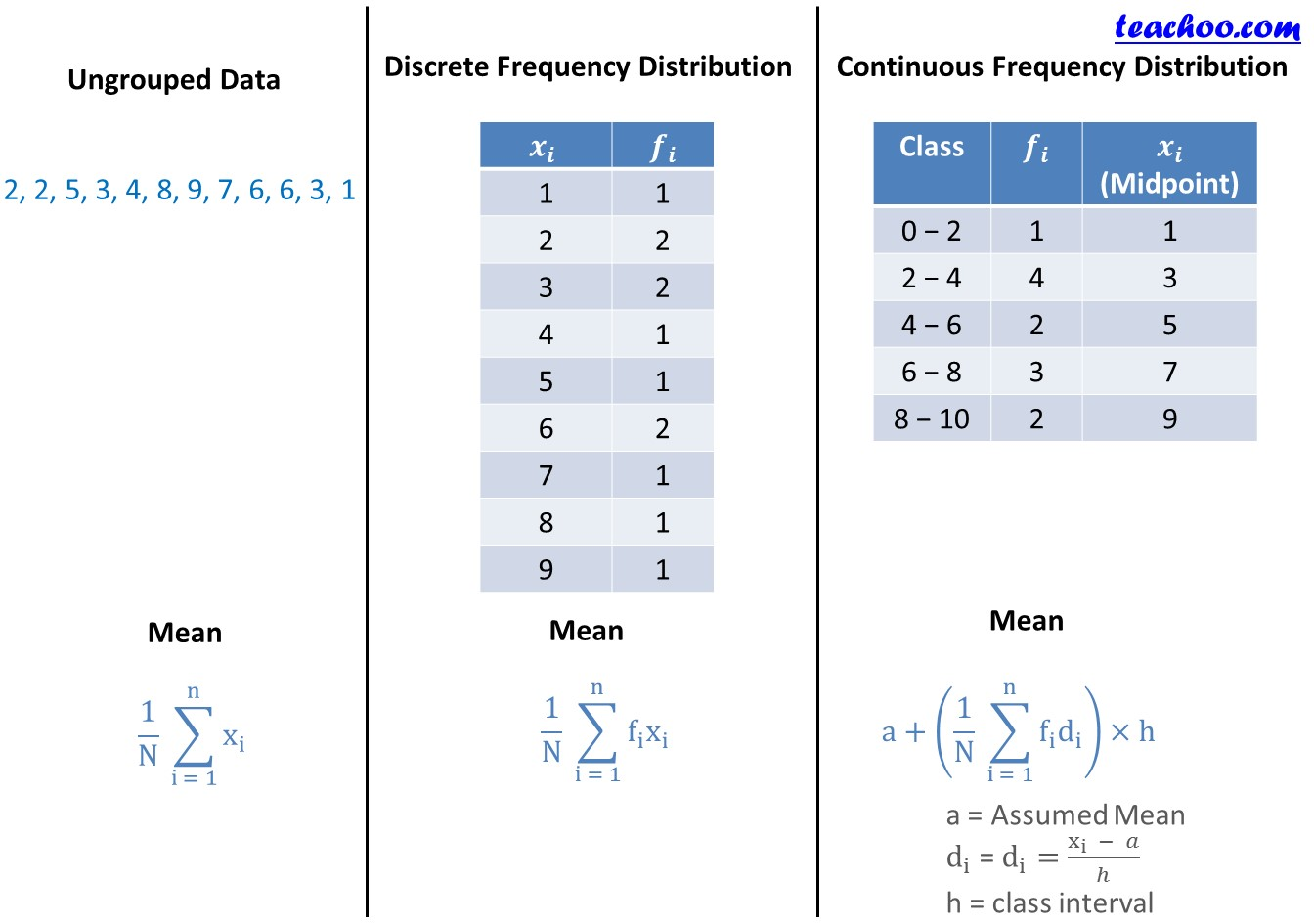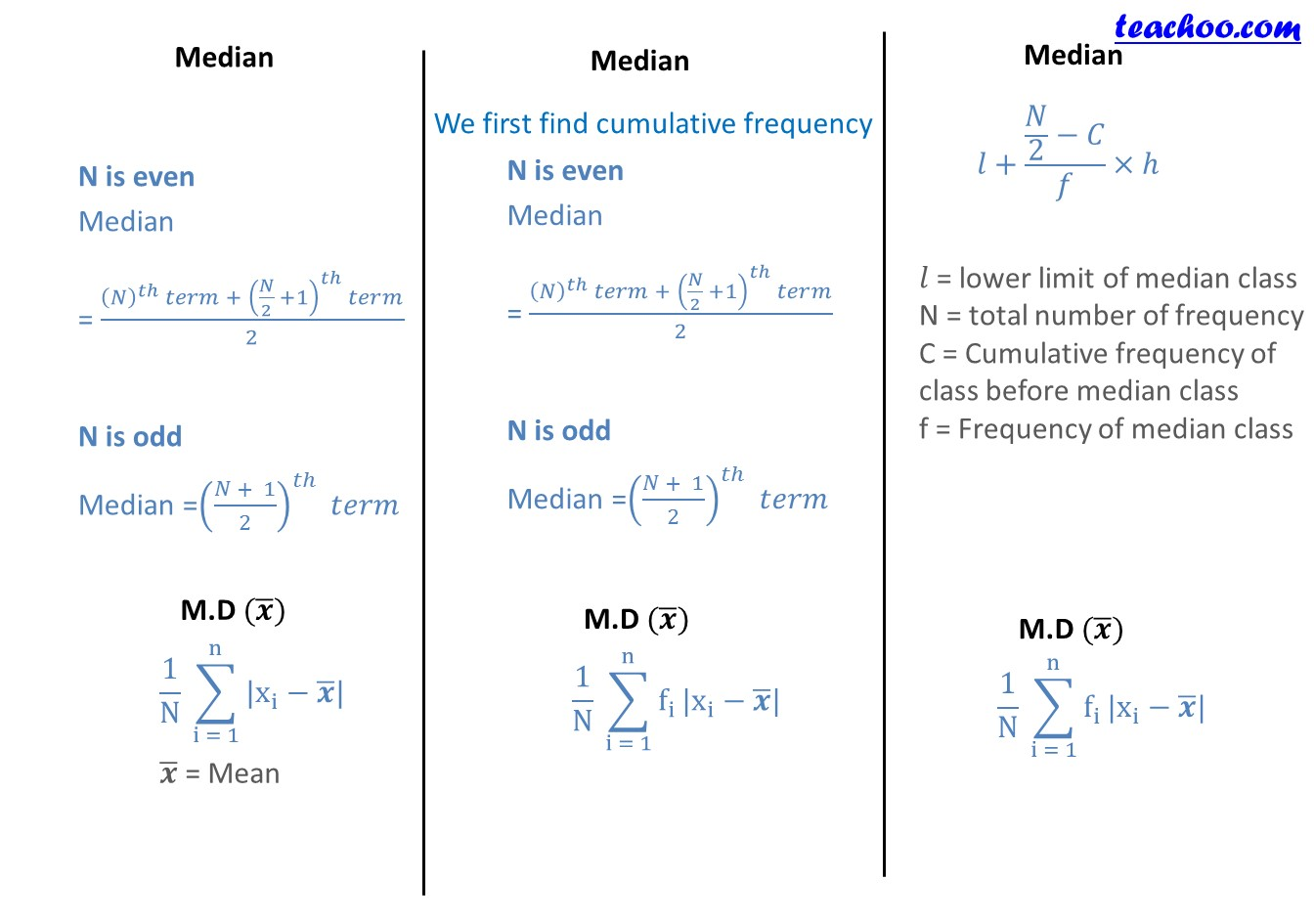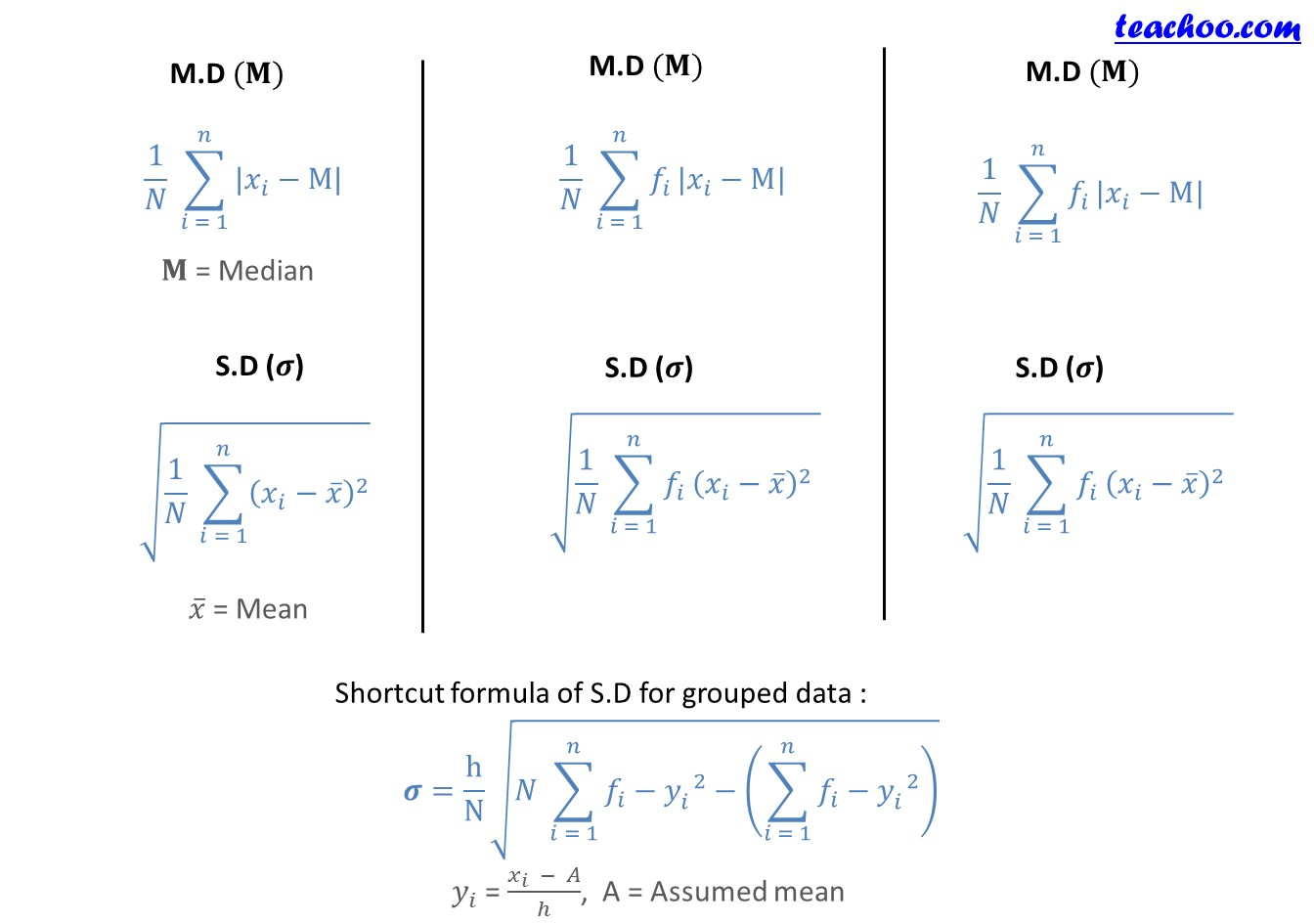Miscellaneous

Chapter 15 Class 11 Statistics
Serial order wise

Check the formula sheet of StatisticsMaths Crash Course - Live lectures + all videos + Real time Doubt solving!

### Transcript

"Chapter 15 Class 11" "Statistics Sheet " "by" teachoo.com Ungrouped Data 2, 2, 5, 3, 4, 8, 9, 7, 6, 6, 3, 1 Mean 1/N ∑24_(i = 1)^n▒x_i Median N is even Median = ((𝑁)^𝑡ℎ 𝑡𝑒𝑟𝑚 + (𝑁/2 +1)^𝑡ℎ 𝑡𝑒𝑟𝑚)/2 N is odd Median =((𝑁 + 1)/2)^𝑡ℎ 𝑡𝑒𝑟𝑚 M.D (𝒙 ̅) 1/N ∑_(i = 1)^n▒〖|x_i−𝒙 ̅|〗 𝒙 ̅ = Mean M.D (𝐌) 1/𝑁 ∑_(𝑖 = 1)^𝑛▒〖|𝑥_𝑖−M|〗 𝐌 = Median S.D (𝝈) √(1/𝑁 ∑_(𝑖 = 1)^𝑛▒(𝑥_𝑖−𝑥 ̅ )^2 ) 𝑥 ̅ = Mean Shortcut formula of S.D for grouped data : 𝝈=h/N √(𝑁 ∑_(𝑖 = 1)^𝑛▒〖𝑓_𝑖−𝑦_𝑖^( 2) 〗−(∑_(𝑖 = 1)^𝑛▒〖𝑓_𝑖−𝑦_𝑖^( 2) 〗) ) 𝑦_𝑖 = (𝑥_𝑖 − 𝐴)/ℎ, A = Assumed mean